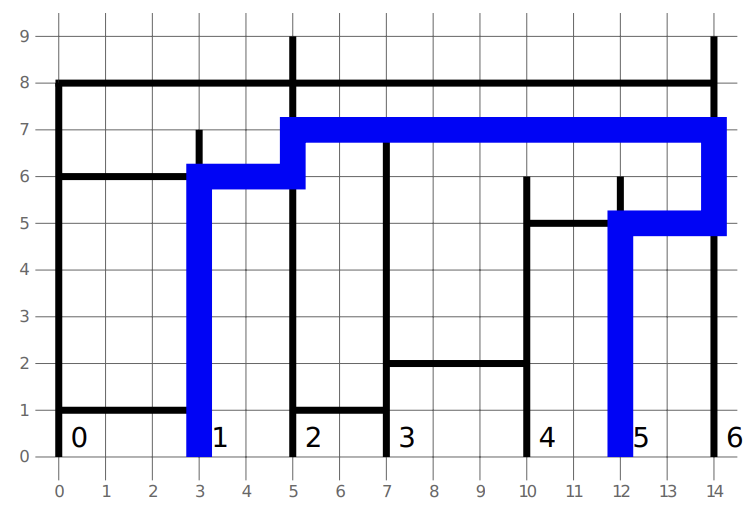시간 제한메모리 제한제출정답맞힌 사람정답 비율
4 초 1024 MB1910952.941%

## 문제

Kenan drew a plan of the buildings and skywalks along one side of the main avenue of Baku. There are $n$ buildings numbered from $0$ to $n-1$ and $m$ skywalks numbered from $0$ to $m-1$. The plan is drawn on a two-dimensional plane, where the buildings and skywalks are vertical and horizontal segments respectively.

The bottom of building $i$ $(0 \leq i \leq n-1)$ is located at point $(x[i], 0)$ and the building has height $h[i]$. Hence, it is a segment connecting the points $(x[i], 0)$ and $(x[i], h[i])$.

Skywalk $j$ $(0 \leq j \leq m-1)$ has endpoints at buildings numbered $l[j]$ and $r[j]$ and has a positive $y$-coordinate $y[j]$. Hence, it is a segment connecting the points $(x[l[j]], y[j])$ and $(x[r[j]], y[j])$.

A skywalk and a building intersect if they share a common point. Hence, a skywalk intersects two buildings at its two endpoints, and may also intersect other buildings in between.

Kenan would like to find the length of the shortest path from the bottom of building $s$ to the bottom of building $g$, assuming that one can only walk along the buildings and skywalks, or determine that no such path exists. Note that it is not allowed to walk on the ground, i.e. along the horizontal line with $y$-coordinate $0$.

One can walk from a skywalk into a building or vice versa at any intersection. If the endpoints of two skywalks are at the same point, one can walk from one skywalk to the other.

## 구현

You should implement the following procedure. It will be called by the grader once for each test case.

int64 min_distance(int[] x, int[] h, int[] l, int[] r, int[] y,
int s, int g)

• $x$ and $h$: integer arrays of length $n$
• $l$, $r$, and $y$: integer arrays of length $m$
• $s$ and $g$: two integers
• This procedure should return the length of the shortest path between the bottom of building $s$ and the bottom of building $g$, if such path exists. Otherwise, it should return $-1$.

## 제한

• $1 \leq n, m \leq 100\,000$
• $0 \leq x < x < \ldots < x[n - 1] \leq 10^9$
• $1 \leq h[i] \leq 10^9$ (for all $0 \leq i \leq n-1$)
• $0 \leq l[j] < r[j] \leq n-1$ (for all $0 \leq j \leq m-1$)
• $1 \leq y[j] \leq \min(h[l[j]], h[r[j]])$ (for all $0 \leq j \leq m-1$)
• $0 \leq s, g \leq n - 1$
• $s \neq g$
• No two skywalks have a common point, except maybe on their endpoints.

## 예시 1

Consider the following call:

min_distance([0, 3, 5, 7, 10, 12, 14],
[8, 7, 9, 7, 6, 6, 9],
[0, 0, 0, 2, 2, 3, 4],
[1, 2, 6, 3, 6, 4, 6],
[1, 6, 8, 1, 7, 2, 5],
1, 5)


The correct answer is $27$.

The figure below corresponds to Example 1:## 예시 2

min_distance([0, 4, 5, 6, 9],
[6, 6, 6, 6, 6],
[3, 1, 0],
[4, 3, 2],
[1, 3, 6],
0, 4)


The correct answer is $21$.

## 서브태스크

번호 배점 제한
1 10

$n, m \leq 50$

2 14

Each skywalk intersects at most 10 buildings.

3 15

$s=0$, $g=n-1$, and all buildings have the same height.

4 18

$s=0$, $g=n-1$

5 43

## 출처• 문제를 만든 사람: Riku Kawasaki

## 제출할 수 있는 언어

C++17, C++14, C++20, C++14 (Clang), C++17 (Clang), C++20 (Clang)

## 채점 및 기타 정보

• 예제는 채점하지 않는다.